# Triangular Prism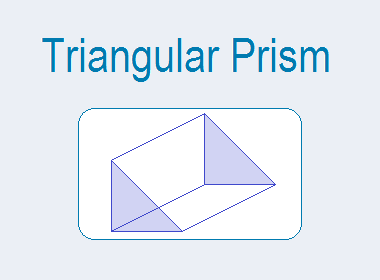## Triangular Prism

A prism having triangular bases is called a triangular prismA triangular prism has three rectangular faces (lateral surfaces) and two triangular faces (bases) as shown in the figure below.

********************

10 Math Problems officially announces the release of Quick Math Solver, an Android App on the Google Play Store for students around the world.

********************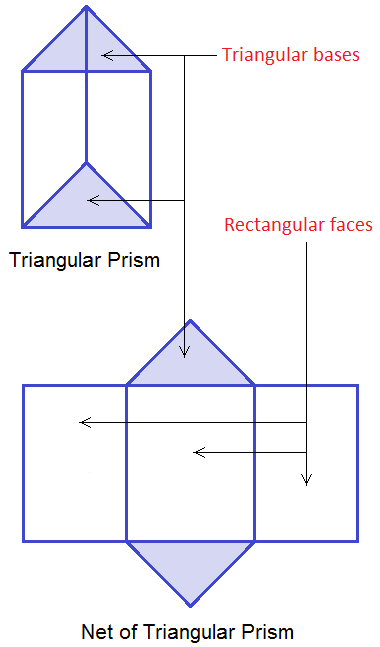Lateral Surface Area of Triangular Prism (LSA)

Let a, b, and c be the three sides of the triangular base, and h be the height of the prism. Lateral surface area of a triangular prism is the area of three rectangular faces (R1, R2 and R3) of the prism as shown in the net of triangular prism in the figure given below.Lateral surface area (LSA)
= area of R1 + R2 + R3

= ah + bh + ch

= (a + b + c)h

= perimeter of triangular base × height

= Ph

[∴ Lateral surface area (LSA) = Ph]

## Total Surface Area of Triangular Prism (TSA)

Total surface area of a triangular prism is the sum of the lateral surface area and the areas of two triangular bases.

Total surface area (TSA)

= LSA + 2 × area of triangular base

= Ph + 2A

[∴ Total surface area (TSA) = Ph + 2A]

## The volume of the Triangular Prism (V)

The volume of a triangular prism is the total space occupied by the prism. It is given by the product of its area of the base and the height of the prism.

i.e. Volume of the triangular prism(V),

= Area of triangular base × height of the prism

= A × h

[∴ Volume of the triangular prism (V) = Ah]

Worked Out Examples

Example 1: Find the lateral surface area, total surface area, and volume of the given triangular prism.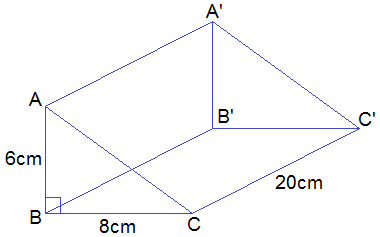Solution:

Here,

Base of the prism is a right-angled triangle,

AB = 6cm

BC = 8cmPerimeter of base (P) = 6 + 8 + 10 =24cm

Area of triangular base (A) = ½ AB × BC

= ½ × 6 × 8

= 24cm2

Height of prism (h) = 20cm

Lateral surface area (LSA) = P × h

= 24cm × 20cm

= 480cm2

Toral surface area (TSA) = LSA + 2A

= 480 + 2 × 24

= 480 + 48

= 528cm2

Volume (V) = A × h

= 24cm2 × 20cm

= 480cm3

LSA = 480 cm2, TSA = 528 cm2 and Volume = 480 cm3  Ans.

Example 2: Find the lateral surface area, total surface area, and volume of the given triangular prism.Solution:

Here,

Base of prism is equilateral triangle of length (a) = 6cm

Perimeter of base (P) = 6 + 6 + 6 =18cmHeight of prism (h) = 15cm

Lateral surface area (LSA) = P × h

= 18cm × 15cm

= 270cm2

Toral surface area (TSA) = LSA + 2A

= 270 + 2 × 15.58

= 270 + 31.16

= 301.16cm2

Volume (V) = A × h

= 15.58cm2 × 15cm

= 233.7cm3

LSA = 270 cm2, TSA = 301.15 cm2 and Volume = 233.7 cm3  Ans.

Example 3: Find the lateral surface area, total surface area, and volume of the given triangular prism.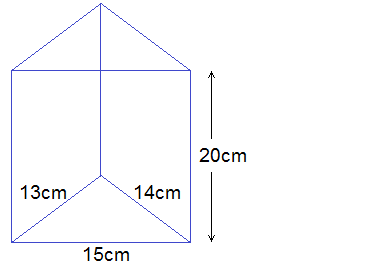Solution:

Here,

Three sides of base triangle are,

a = 13cm

b = 14cm

c = 15cm

Perimeter of base (P) = 13 + 14 + 15 = 42cm

Semi-perimeter (s) = 42/2 = 21cm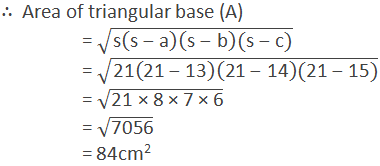Height of prism (h) = 20cm

Lateral surface area (LSA) = P × h

= 42cm × 20cm

= 840cm2

Toral surface area (TSA) = LSA + 2A

= 840 + 2 × 84

= 840 + 168

= 1008cm2

Volume (V) = A × h

= 84cm2 × 20cm

= 1680cm3

LSA = 840 cm2, TSA = 1008 cm2 and Volume = 1680 cm3  Ans.

If you have any question or problems regarding the Triangular Prism, you can ask here, in the comment section below.